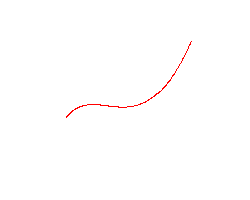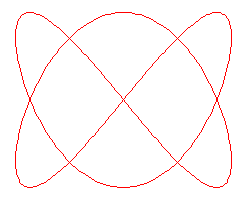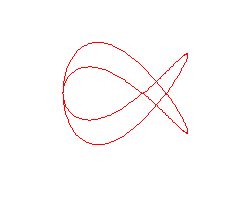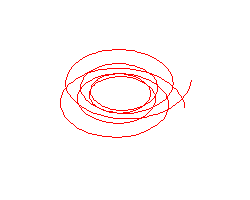﻿ ﻿ math handbook calculator - Fractional Calculus Computer Algebra System software+++=# Example of Math Graphics 数学函数图 制图法

## Space curve

A simple straightforward way to run interactive mathematics in a web page is by mathHand.com. All 3D graphes can be zoom, move or spin. One variable for space curve, and two variables for space surface.

### by parametric3D(x)

1. ellipsoid
2. conjugate-roots

#### 3 equations with 1 variable t or x

3. Cabello's Curve 曲线
4. parametric3D(cos(t),sin(t),t)
5. parametric3D(sin(x),cos(x),x)
6. parametric3D(x,x,x)

#### 2 equations with 1 variable t or x

the 2D curve becomes 3D by clicking the 3D checkbox
7. parametric3D(x,x)
8. complex sn(x) Curve

### by wireframe3D(x)

9. wireframe3D(x)
10. wireframe3D(sin(x),cos(x),x)

### by spin3D(x)

spin different directions by clicking the spin checkbox.
11. spin3D(x**2)

#### Animation

The parametric3D( ) plots animation by clicking the spin checkbox.
12.A space cubic curve. Most simple, but only its movement shows what it is about.
13.A Lissajous figure with rotating phase.
14.The above Lissajous figure is a projection of this space curve.
15.Another space curve, a little bit more complicated: hyperbolic spiral.

### Reference 参阅

• example 例题
• graph 制图
• graphics 制图法
• animation 动画 ﻿